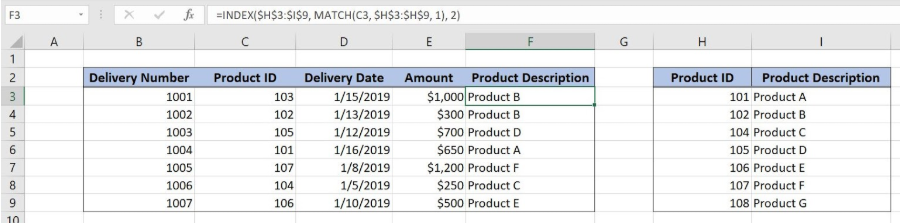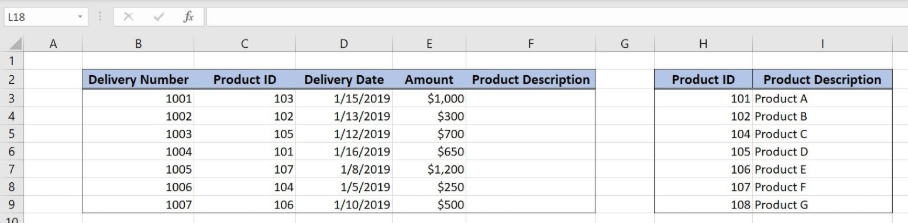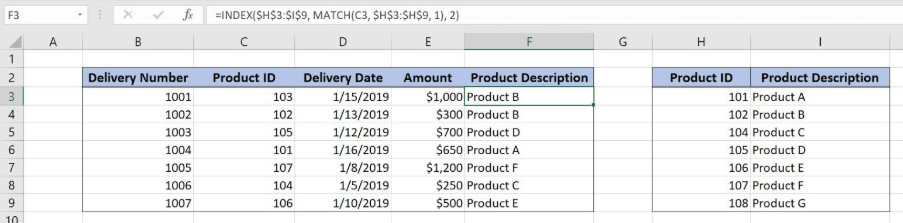Get instant live expert help with Excel or Google Sheets“My Excelchat expert helped me in less than 20 minutes, saving me what would have been 5 hours of work!”

#### Post your problem and you’ll get expert help in seconds.

Your message must be at least 40 characters
Our professional experts are available now. Your privacy is guaranteed.

# Learn How to Perform Approximate Match in Excel

Excel allows us to lookup values using an approximate match with INDEX and MATCH functions. The MATCH function returns a row for a value in a table, while the INDEX returns a value for that row. This step by step tutorial will assist all levels of Excel users to learn how to perform an approximate match in Excel.Figure 1. The final result of the formula

## Syntax of the INDEX formula

The generic formula for the INDEX function is:

`=INDEX(array, row_num, column_num)`

The parameters of the INDEX function are:

• array – a range of cells where we want to get a data
• row_num – a number of a row in the array for which we want to get a value
• column_num – a column in the array which returns a value.

## Syntax of the MATCH formula

The generic formula for the MATCH function is:

`=MATCH(lookup_value, lookup_array, [match_type])`

The parameters of the MATCH function are:

• lookup_value – a value which we want to find in the lookup_array
• lookup_array – the array where we want to find a value
• [match_type] – a type of match. We put 1 as an approximate match. If the exact match is not found the first value less than lookup_value is returned.

## Setting up Our Data for the Formula

Our table consists of 5 columns: “Delivery Number” (column B), “Product ID” (column C), “Delivery Date” (column D), “Amount” (column E) and “Product Description” (column F).

In column F, we want to populate a Product Description from the Lookup table in the range H3:I9. This table consists of 2 columns: “Product ID” (column H) and “Product Description” (column I). Two tables are joined by the columns “Customer ID”.Figure 2. Data that we will use in the example

## Performing an Approximate Match Using the INDEX and MATCH Formula

We want to get a product description in the cell F3, from the lookup table H3:I9, based on the Product ID 103 in the cell C3. If Product ID 103 is not found in the lookup table, we want to the first closest value less than 103. In our case, it is 102.

The formula looks like:

`=INDEX(\$H\$3:\$I\$9, MATCH(C3, \$H\$3:\$H\$9, 1), 2)`

The lookup_value parameter of the MATCH function is C3. The lookup_array is \$H\$3:\$H\$9, while the match_type is 1, which means the approximate match. The result of the MATCH function is the row_num parameter of the INDEX function. The array the range \$H\$3:\$I\$9.

To apply the formula, we need to follow these steps:

• Select cell F3 and click on it
• Insert the formula: `=INDEX(\$H\$3:\$I\$9, MATCH(C3, \$H\$3:\$H\$9, 1), 2)`
• Press enter
• Drag the formula down to the other cells in the column by clicking and dragging the little “+” icon at the bottom-right of the cell.Figure 3. Using the INDEX and MATCH Formula

The MATCH function will return 2, as Product ID 103 is not in the lookup table H3:I9. The closest value less than 103 is 102, in row 2. Therefore, the INDEX function returns the Product description from the second row. The result in the F3 is “Product B”.

Most of the time, the problem you will need to solve will be more complex than a simple application of a formula or function. If you want to save hours of research and frustration, try our live Excelchat service! Our Excel Experts are available 24/7 to answer any Excel question you may have. We guarantee a connection within 30 seconds and a customized solution within 20 minutes.

Solution examplesindex and match with duplicates. I need to use another column as a reference, so my return value has two match the value of two things for it to return
Solved by E. H. in 60 minsHello, I have a big spreadsheet in which I need to know how many patients came in each month based on provider. I am using the following formula but I still get the N/A error. =INDEX('No Show Appts Data'!D:E, MATCH(1, 'No Show Appts Data'!D:D='No Shows Data'!M2)*('No Shows Data'!E:E='No Shows Data'!N1),0)) No Show Appts Data is the name of the sheet where Column D is Month Year of date in question and Column E is the provider. No Shows Data is the sheet where I am making all the formula calculation where Column M is Month and Year and Column N is the provider in question and therefore M2 is the month in question and N1 the provider in question. How do I fix this error? Thanks
Solved by F. H. in 40 minsI need to find an INDEX function that will convert the Call Day (a number 1-7) to the actual weekday found in row 1 of the DayofWeek named range. I don't know if I'm supposed to use the MATCH function as well or not.
Solved by D. D. in 11 minsI have two columns with names and a third one with email addresses. For every name that matches, I need to copy the email address to an empty column next to the matching name. .
Solved by E. U. in 15 minshelp with INDEX(IndexArea,MATCH(\$M\$5,MatchRows,0),MATCH(\$M\$6,MatchColumns,0))
Solved by Z. Y. in 12 mins